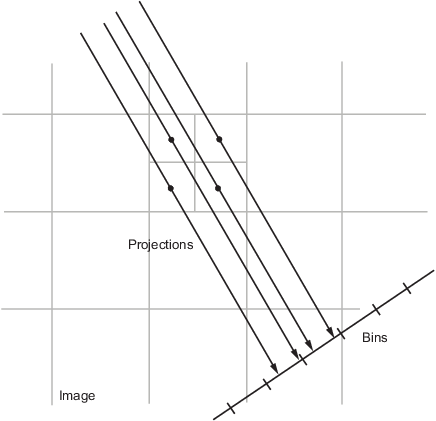## 语法

R = radon(I)
R = radon(I,theta)
[R,xp] = radon(___)

## 说明

R = radon(I) 返回二维灰度图像 I 的 Radon 变换 R，角度范围为 [0, 179] 度。Radon 变换是图像强度沿特定角度的径向线的投影。

R = radon(I,theta) 返回基于 theta 所指定角度的 Radon 变换。
[R,xp] = radon(___) 返回向量 xp，其中包含与图像的每行对应的径向坐标。

## 示例

iptsetpref('ImshowAxesVisible','on')

I = zeros(100,100); I(25:75, 25:75) = 1;

theta = 0:180; [R,xp] = radon(I,theta);

imshow(R,[],'Xdata',theta,'Ydata',xp,'InitialMagnification','fit') xlabel('\theta (degrees)') ylabel('x''') colormap(gca,hot), colorbariptsetpref('ImshowAxesVisible','off')

## 输出参数

• 如果 theta 是标量，则 R 是数值列向量，其中包含基于 theta 度的 Radon 变换。

• 如果 theta 是向量，则 R 是矩阵，其中每列是基于 theta 中某一角度的 Radon 变换。

floor((size(I)+1)/2)

## 算法Bracewell, Ronald N., Two-Dimensional Imaging, Englewood Cliffs, NJ, Prentice Hall, 1995, pp. 505-537.

 Lim, Jae S., Two-Dimensional Signal and Image Processing, Englewood Cliffs, NJ, Prentice Hall, 1990, pp. 42-45.